Search

Solving Quadratic Inequalities: Concepts (page 1 of 3)Solving linear inequalities, such as "x + 3 > 0", was pretty straightforward, as long as you remembered to flip the inequality sign whenever you multiplied or divided through by a negative (as you would when solving something like "2x < 4").

There is a big jump, though, between linear inequalities and quadratic inequalities. Part of the jump is the fact that concepts which were skipped over in learning how to solve linear inequalities are useful, even needful, in solving quadratic inequalities. So let's first look at a linear inequality, and cover those concepts that were skipped earlier.

• Solve x  4 < 0.
• I already know that, to solve this inequality, all I have to do is add the 4 to the other side to get the solution "x < 4". So I already know what the answer is. But now I'll approach this problem from a different angle, by considering the related two-variable graph.

 For "x  4 < 0", the associated two-variable linear graph is y = x  4: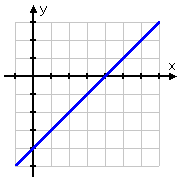The inequality "x  4 < 0" is asking "when is the line y = x  4 below the line y = 0?" Since the line y = 0 is just the x-axis, the inequality is therefore asking "when is the line y = x  4 below the x-axis?" The first step in answering this question is to find where the line crosses the x-axis; that is, first I need to find the x-intercept. So I set y equal to zero and solve:

y = x  4
0 = x  4

4 = x

So the line y = x  4 crosses the x-axis at x = 4. Since the line y = x  4 is a straight line, it will be above the x-axis on one side of the intercept and below the x-axis on the other side of the intercept.   Copyright © Elizabeth Stapel 2002-2011 All Rights Reserved

 Since the slope of this line is m = 1 (in particular, since the slope is positive), then the line is increasing, so the line is below the axis on the left-hand side (before the intercept) and above the axis on the right-hand side (after the intercept), as is highlighted at right: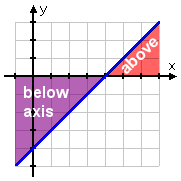The original question asked me to solve x  4 < 0, so I need to find where the line is below the x-axis. This happens on the left-hand side of the intercept: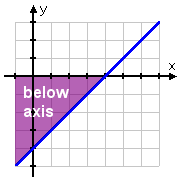Since the original inequality, "x  4 < 0", asked only about the x-values, I'll restrict the above graph to just the x-axis: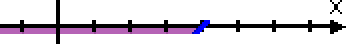Thinking back to the graphical method of presenting solutions to linear inequalities, the above graph displays the correct solution "x < 4".

That is, by looking at the graph of the associated line and determining where (on the x-axis) the graphed line was below the x-axis, you can easily see that the solution to the inequality "x  4 < 0" is the inequality "x < 4". You can follow the same method of finding intercepts and using graphs to solve inequalities containing quadratics.

Let's look at a quadratic inequality:

• Solve x2 + 4 < 0.

First, I need to look at the associated two-variable equation, y = x2 + 4, and consider where its graph is below the x-axis. To do this, I need to know where the graph crosses the x-axis. That is, I first need to find where x2 + 4 is equal to zero:

x2 + 4 = 0
x2  4 = 0

(x + 2)(x  2) = 0

x = 2
or  x = 2

This says that the quadratic crosses the x-axis at x = 2 and at x = 2.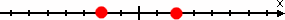These zeroes divide the number line into three intervals: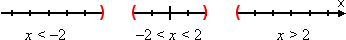Now I need to figure out where (that is, on which intervals) the graph is below the axis. But that's easy! Since this is a "negative" quadratic, it graphs as an upside-down parabola.

 In other words, the graph is high (above the axis) in the middle, and low (below the axis) on the ends: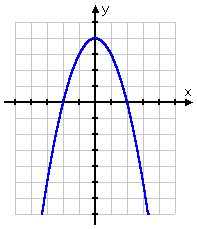To solve the original inequality, I need to find the intervals where the graph is below the axis (so the y-values are less than zero). My knowledge of graphing, together with the zeroes I found above, tells me that that I want the intervals on either end, rather than the interval in the middle: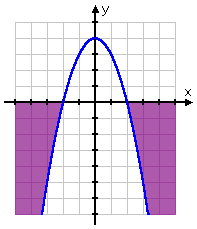Then the solution is clearly:

x < 2 or x > 2

I could have multiplied the initial inequality through by 1, giving me "x2  4 > 0". The zeroes would have been the same: x = 2 and x = 2. But this parabola would have been right-side-up, since the quadratic would have been "positive". That's okay though, because, by multiplying through by 1, I would have flipped the inquality, so I would have been looking for where the quadratic is greater than zero (that is, where the parabola is above the axis). Since the parabola would have been right-side-up, the graph would have been above the axes on the ends; so the solution would have worked out to be the same as before: x < 2 or x > 2: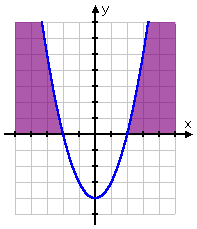Top  |  1 | 2 | 3  |  Return to Index  Next >>